• Call Now

1800-102-2727•

# Friction On Inclined Plane: Explanation, Examples, Motion of Objects on Inclined Plane

While walking on an inclined surface you must have observed that it’s harder to walk on the steep surface than horizontal surface. While riding a bike if you stop on a steep road sometimes it becomes very hard to stand on that steep road. This is because while you are on a steep surface the value of normal force decreases as the steepness increases. So as the normal force decreases the frictional force also decreases. In this article we will study all about the friction on an inclined plane.

• Friction on inclined plane
• Block over block system
• Practice problems
• FAQs

## Friction on Inclined Plane

Depending on the motion of the block on an inclined plane the value of frictional force and its direction is decided. Let’s understand it case by case:

Case1: Object at rest on inclined planeWhen an object is at rest on an inclined plane as shown in the figure the force of friction is static in nature. The direction of static friction is in upward direction as the block has the tendency to move downwards.

In this case,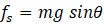Case2: Object is moving upwards on inclined planeAs there is relative slipping between the surfaces the force of friction is kinetic in nature. As an object is moving upwards the direction of friction will be in downward direction as shown in the figure.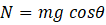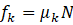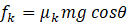Case3: Object is moving downwards on inclined planeAs there is relative slipping between the surfaces the force of friction is kinetic in nature. As an object is moving downwards the direction of friction will be in upward direction as shown in the figure.## Block Over Block System

Let’s take an example to understand this type of problem,

All surfaces shown are rough. Draw the kinetic friction force on 𝐴 and 𝐵.Solution: Block 𝐴 is in contact with only block B. Hence, there is only one kinetic frictional force acting on block ??. While block B is in contact with block 𝐴 and the ground. Hence, there are two kinetic frictional forces acting on block B

Direction of kinetic friction force on 𝐴 exerted by B

Kinetic friction acts opposite to the relative velocity of the object with respect to the other object in contact.

To find relative velocity of 𝐴 with respect to B,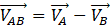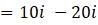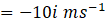Relative velocity of 𝐴 with respect to B is in the negative x direction. So, the direction of kinetic friction exerted on 𝐴 by B is in the positive x direction.

Direction of kinetic friction force on B exerted by A

To find relative velocity of B with respect to 𝐴, take 𝐴 as the frame of reference.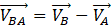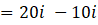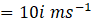Relative velocity of B with respect to 𝐴 is in positive x direction. So, the kinetic friction force on B is acting in negative x direction.

Kinetic friction on B exerted by ground

To find the relative velocity of B with respect to the ground, take ground as the frame of reference.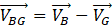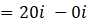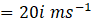Relative velocity of B with respect to the ground is in the positive x direction. So, the direction of kinetic friction on B exerted by ground is in the negative x direction.

Video Explanation: two block problems (time stamp: 1.02.00 to 1.21.50)

## Practice problems

1. For the given situation, what would be the acceleration of the block and the magnitude of the friction acting on the block?Solution:

From the FBD of the block,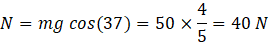Force causing the motion=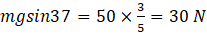Limiting friction,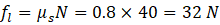Since, force causing motion is less than limiting friction then friction is static

So,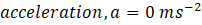.

Static friction force acting on block =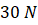2.  For the given situation, what would be the acceleration of the block and the magnitude of the friction acting on the block?Solution: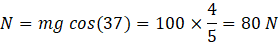Force causing motion =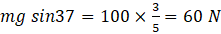Limiting friction,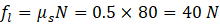As force causing motion is greater than limiting friction then its kinetic friction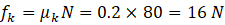Acceleration,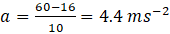.

3.  A block of mass 2 kg is placed on a fixed inclined plane as shown in the figure. The angle of inclination of the plane is 𝜃 = 30°. Find the minimum value of F such that the block does not slide?Solution: When the minimum force required for equilibrium is applied, then the limiting frictional force acts between the block and the inclined plane.

FBD of the block is,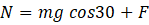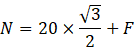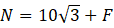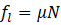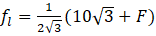……….(1)

As the block is in equilibrium,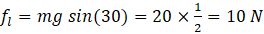……(2)

Equating equation (1) and (2),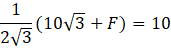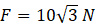4.  A body of mass m just starts sliding down on the inclined plane when ∠BAC = 300 as shown in the figure. Find the coefficient of friction?Solution: Angle of repose is the angle (𝜃) made by an inclined plane with the horizontal such that an object placed on the inclined surface just begins to slide.

Tangent angle of repose gives the coefficient of friction.

Angle of repose,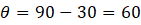Coefficient of friction,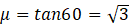5. A variable force F =10 t is applied to block B placed on a smooth surface. The coefficient of friction between A and B is 0.5. (t is time in seconds. Initial velocities are zero). At what instant block A starts sliding on B?A. Maximum friction block A is,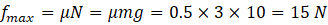Block A will start sliding when friction on it will become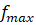So maximum acceleration with which two block will move together is,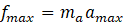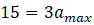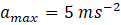Applying Newton’s law on block B at this instant,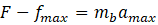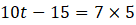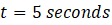## FAQs

1. What is friction?
Solution:
Friction is a force related to two surfaces in contact, which opposes the relative motion between them.

2. What is the direction of kinetic friction?
Solution:
Kinetic friction opposes the relative motion between objects. So, for an object, the direction of kinetic friction is opposite to its relative velocity with respect to the other object in contact.

3. What is the direction of friction on the object which is at rest on the inclined plane?
Solution:
Object has the tendency to move downwards when kept on an inclined plane then the direction of friction is upwards.

4. What is the angle of repose?
Solution:
It is the minimum angle made by an inclined plane with the horizontal such that an object placed on the inclined surface just begins to slide.Talk to our expert
Resend OTP Timer =
By submitting up, I agree to receive all the Whatsapp communication on my registered number and Aakash terms and conditions and privacy policy